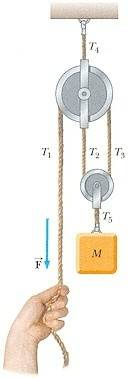# Force and Pulley Problem (Pic Included)

## Homework Statement

A mass M is held in place by an applied force , FA , and the pulley system shown in the figure below. The pulleys are massless and frictionless. Express the tension in each section of the rope, FT1, FT2, FT3, FT4, and FT5, as well as the applied force, FA , in terms of M and g.

Here is a picture I found online:F=ma

## The Attempt at a Solution

I looked at the picture and saw that the force being applied by mass M was split in half because of the strings separating. I then came to conclusion that FT1 must be FT1=mg/2, because the force of mass M was cut in half(I think this is correct).

I then looked at FT4 and knew that it was the addition of FT1+FT5. The masses force was split in half like i said earlier, so now FT2+FT3+FT1=FT4. mg/2+mg/2+mg/2= 3mg/2. So the force on FT4 is 3mg/2 (I think this is the correct answer).

FT5 is just the force of mass M pulling down, so FT5=mg

To wrap it all up these are my answers:
FT1=mg/2
FT2=mg/2
FT3=mg/2
FT4=3mg/2
FT5=mg
FA=FT1=mg/2

I just want to see if my thought process is right on these and if my answers are correct. We have not gotten into pulleys yet so it was somewhat of an educated guess.

Last edited:

tiny-tim
Homework Helper
Hi Dcarroll!I looked at the picture and saw that the force being applied by mass M was split in half because of the strings separating. I then came to conclusion that FT1 must be FT1=mg/2, because the force of mass M was cut in half(I think this is correct).

I then looked at FT4 and knew that it was the addition of FT1+FT5. The masses force was split in half like i said earlier, so now FT2+FT3+FT1=FT4. mg/2+mg/2+mg/2= 3mg/2. So the force on FT4 is 3mg/2 (I think this is the correct answer).

FT5 is just the force of mass M pulling down, so FT5=mg

To wrap it all up these are my answers:
FT1=mg/2
FT2=mg/2
FT3=mg/2
FT4=3mg/2
FT5=mg
FA=FT1=mg/2

Yes, they're all correct.But I don't like your "cut in half" argument …

the correct method is to say "consider the forces on the small (masless) pulley" … they are T2 + T3 up, and T5 down; and T2 = T3, and the acceleration is zero, so 2T3 - T5 = 0

similarly, consider the forces on the large pulley, and the forces on the mass MAhh ok thanks a bunch, like i said if never done pulleys so the correct way to do things helps lol